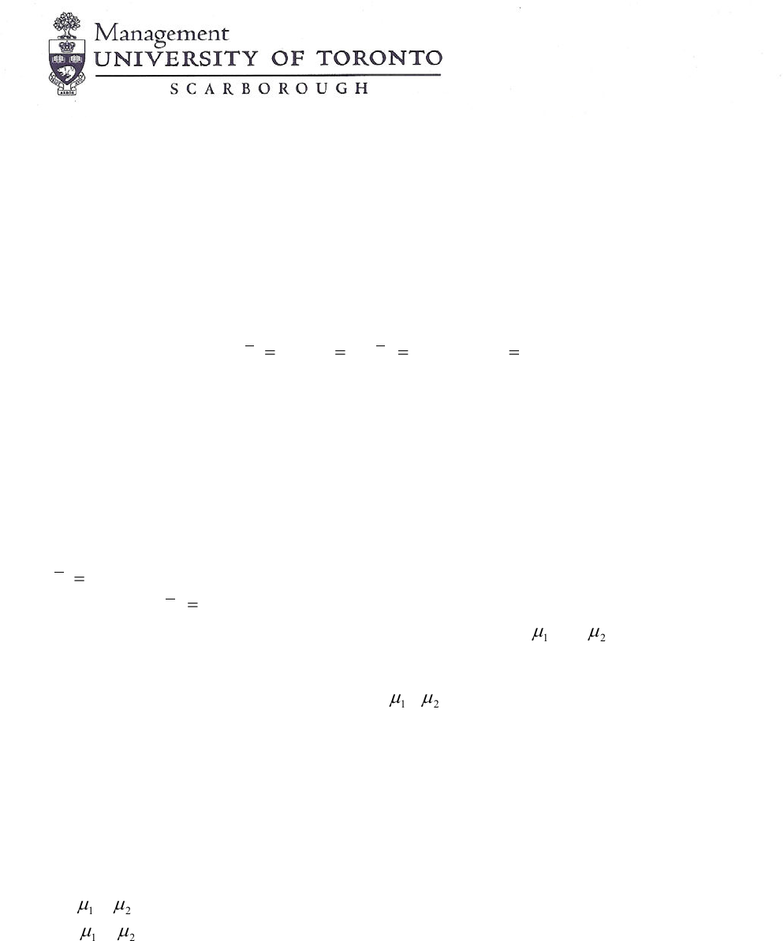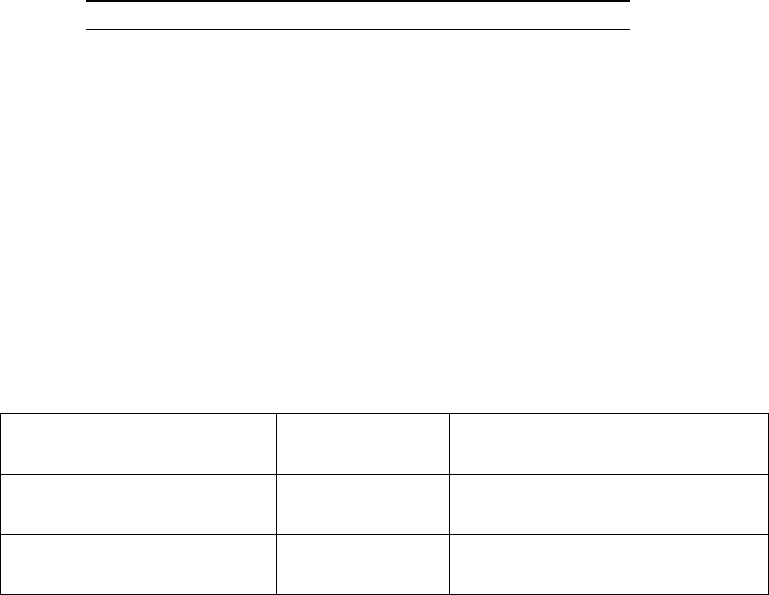Class Notes (1,100,000)
CA (650,000)
UTSC (30,000)
ATA (10)
Lecture 3

# MGEB12H3 Lecture Notes - Lecture 3: Standard Deviation, Confidence Interval, Test StatisticExam

Department
Economics for Management Studies
Course Code
MGEB12H3
Professor
ATA
Lecture
3

This preview shows pages 1-3. to view the full 11 pages of the document.MGEB12: Quantitative Methods in Economics-II
Problem Set-3
Chpater-10: 36, 2, 4, 8, 10, 14
Supplemental:
1. You are interested in testing whether two groups of students perform identically. Two random samples of 80
students were drawn independently from two normal populations. The following statistics regarding their scores
in a final exam were obtained:
74
1=x
,
8
1
=s
,
72
2=x
, and
5.6
2=s
. The p-value for your test is
approximately equal to:
A. 0.0324
B. 0.0443
C. 0.0658
D. 0.0771
E. 0.0827
Questions 2-4: You are interested to compare the effect of two workouts on heart. 50 adult volunteers were
randomly divided into two groups of 25 subjects each. Group A took the workout A and recorded a mean heart
rate of
90
1=x
per minute with a standard deviation of s1 = 9 beats per minute. Group B took workout B and
recorded mean heart of
95
2=x
beats per minute with a standard deviation s2 = 12 beats per minute. Assume the
two groups are independent and the data are approximately normal. Let
1
µ
and
2
µ
represent the true mean
heart rates using two workouts.
2. What is a 95% confidence interval for the difference
1
µ
-
?
A. -5 ± 6.04 beats per minute
B. 5 ± 6.04 beats per minute
C. -5 ± 5.13 beats per minute
D. 5 ± 5.13 beats per minute
E. 5 ± 7.12 beats per minute
3. Using the above data, if you wished to test the hypotheses:
Ho:
1
µ
=
Ha:
1
µ
<
The P-value for the test would be: (Use the conservative value for the degrees of freedom.)
A. larger than .10
B. between .10 and .05
C. between .05 and .01
D. between .01 and 0.005
E. below 0.005

Only pages 1-3 are available for preview. Some parts have been intentionally blurred.

2
4. At what level of significance do the above data provide enough evidence to reject the null hypothesis?
A. lower than 1%
B. 1%
C. 5%
D. 6%
E. 10%
Questions 5-7: An SRS (Solar Road Stud) of 100 flights of airline A recorded 64 on time. An SRS of 100
flights of airline B recorded 80 were on time. If p1 is the proportion of all flights that are on time for airline A
and p2 is the proportion of all flights that are on time for airline B.
5. The 95% confidence interval for the difference p1 – p2 is:
A. -0.16 ± 0.122
B. -0.16 ± 0.062
C. 0.16 ± 0.062
D. -0.16 ± 0.103
E. -0.16 ± 0.114
6. Using the above data, you are interested in testing if there is evidence of difference in the on-time rate for the
two airlines? To determine this, you are to evaluate the following hypotheses:
Ho: p1 = p2
H1: p1 # p2
The P-value of your test is:
A. between .05 and .01
B. between .10 and .05
C. below .001
D. between .01 and .001
E. greater than 0.10
7. At what level of significance do the above data provide enough evidence to reject the null hypothesis?
A. 10%
B. 5%
C. 2%
D. all of the above levels
E. none of the above
8. A researcher believes that the proportion of women who like chocolate is less than the proportion of men
who like chocolate. To find out she asks 120 women if they like chocolate, and 102 say they do. Then she asks
150 men if they like chocolate, and only 132 of them do. The p-value of the test is approximately:
A. .0612
B. .1534
C. .2358
D. .3212
E. .4651

Only pages 1-3 are available for preview. Some parts have been intentionally blurred.3
9. A truck manufacturer has two plants. The CEO of this company suspects that plant –1 is more efficient (in
terms of number of trucks produced each month) than the plant –2. Monthly production data was collected from
both plants and reported in the following table:
Plant-1
Plant-2
Mean
57.75
55.67
Variance
5.84
13.15
Observations
12
12
Based on this, the p-value of the hypothesis is: (Assume equal variances)
A. p-value < 0.01
B. 0.010 < p-value < 0.025
C. 0.025 < p-value < 0.050
D. 0.050 < p-value < 0.100
E. p-value > 0.10
Questions 10-11: A university is deciding whether to require students to take quantitative methods. It randomly
samples graduates and asks “Are you satisfied with your career options?” Here is a summary the data:
Took
quantitative methods 56 75%
Did not take quantitative
methods 136 64%
10. The university wants to know if the difference in satisfaction is statistically significant. What is the test
statistic?
A. 1.48
B. 1.57
C. 1.66
D. 1.98
E. 2.18
11. Does this test of statistical significance inform the decision about whether to require quantitative methods?
A. Yes, because we found a statistically significant result
B. Yes, because we can infer that training in quantitative methods increases satisfaction
C. No, because we did not find a statistically significant result
D. No, because these data are experimental and we cannot infer that training in quantitative methods increases
satisfaction
E. No, because these data are observational and we cannot infer that training in quantitative methods increases
satisfaction
Questions 12-14: You’re a financial analyst for a brokerage firm. You are attempting to test if companies listed
on NYSE pay different dividend yield than those listed on NASDAQ. You collect the following data:
Group N Mean Standard Deviation
NYSE 21 3.27 1.30
NASDAQ 25 2.53 1.16